# Kissing the frog: A mathematician's guide to mating

September 2008

### Finding the Decision Numbers

As discussed in the main article, if the frogs are randomly numbered between 0 and 1, with the prince having the highest number, there is a sequence of decision numbers associated with the best strategy for choosing the top frog. Since we're meant to be doing some maths, we need some notation. We'll consider the general case with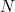frogs, and let the number on the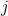th frog be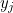,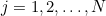. In addition, we'll try to find an increasing sequence of decision numbers,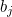,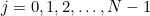, such that we kiss theth frog if it has the largest number seen so far and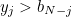. In other words, to think about kissing the last frog we need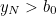, the next to last frog,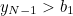, and so on. The reasons for this back to front numbering of the decision numbers should become clear below.

Let's assume that the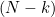th frog has the largest number so far on its back,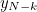, which we'll write as just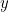to make things look nicer on the page. There are therefore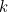frogs still to come out of the pond. If we kiss the frog, the probability that he's the handsome prince is equal to the probability that the remainingfrogs have numbers less than, which is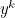. That's the easy part of the calculation. The next bit is rather more tricky, but basically just involves counting (or combinatorics as it's rather grandly called by mathematicians).

If we don't kiss the frog, but choose the next one with number larger than, we can work out the probability that we kiss the prince by considering the situation when there are exactlyfrogs remaining that have numbers higher than the current frog's,, and adding up our answers for all possible values of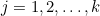. The probability that there are exactlyfrogs remaining that are numbered higher thanis equal to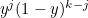times the number of different ways that we can choosefrogs from the final, which is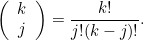The probability that the prince comes first in the sequence ofhigher-numbered frogs, which is the only position in which he can get kissed, is therefore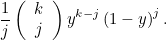The appropriate decision number for the current frog is the value ofat which the probability of winning by kissing and the probability of winning by not kissing are equal. This gives us, remembering that we must sum over all possible values of,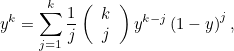or, by defining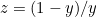,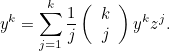Bringing everything over to the right hand side and factoring out thegives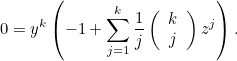Sincecannot possibly be zero, this leads to the equation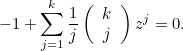(1)
Once we've found the unique positive solution of this,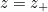(can you prove there's one and only one positive solution?), theth decision number is given by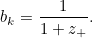(2)
Let's try this for various values of. Note that if you're up to the last frog, you'd obviously kiss it if it's the highest so far, so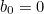. If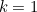there's just one frog remaining after the current frog. The average value of this final frog is 0.5, so we should kiss the last but one frog if it's the highest we've seen so far and its number satisfies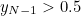. We therefore expect that the corresponding decision number is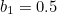. Now, with, equation (1) becomes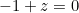, with the pretty obvious positive solution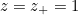, and hence from equation (2),, as expected. When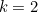, there are two frogs remaining, and the value of the decision number is less obvious. Equation (1) becomes, after rearranging, the quadratic equation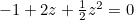, which has a positive solution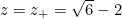, and hence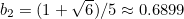. You should be able to see these last three decision numbers,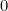,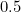,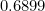, on the graphs plotted in the main article. We need to solve a cubic equation to find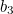, a quartic equation to find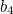, and so on. Pencil and paper isn't much help here but, because equation (1) has a unique positive solution, it's straightforward to find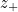iteratively using Newton's method. You can see the first 100 decision numbers in the graphs in the main article. One other thing we'd like to know is how the decision numbers behave asgets large. The maths gets a little harder here, so if you've had enough, you can return to the main article.

### What happens as k gets large?

We can see from the graphs in the main article that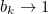as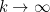, but how fast does this happen? In order to do this, we need to find the positive solution of equation (1) whenis large. You could have a think about how you might do this before reading on, but I'll warn you that it's not easy. The summed part of equation (1) looks like a binomial series, except for the factor of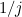multiplying the terms. However, if we differentiate the series, this goes away. This suggests that we can write the equation as an integral of a binomial series, and a little experimentation shows that equation (1) is equivalent to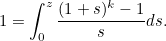(3)
If you don't believe me, use the binomial theorem on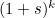and then integrate the series you get term by term. How does equation (3) help us? Well, integrals are always easier to estimate than sums, and we need to estimate the integral in equation (3) whenis large. In general,will be large whenis large, but the left hand side of the equation is just 1. Let's look for a solution with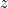, and therefore, small. If we define new variables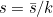and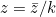, equation (3) becomes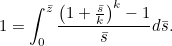(4)
Now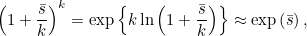whenis large. We have used the first term in the Taylor series expansion of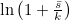to approximate it here. We can therefore write (4) as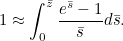(5)
This is now just one equation that we must solve, since we've managed to remove. We can solve this on a computer, approximating the integral using, for example, the trapezium rule, and find the solution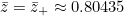. We now just have to work our way back through our changes of variable; first,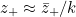, and then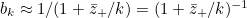, and hence, applying the binomial theorem to this final result,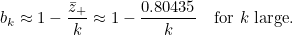We have therefore shown that the decision numbers do, as expected, approach 1 from below asgets large, decaying algebraically, like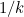as.